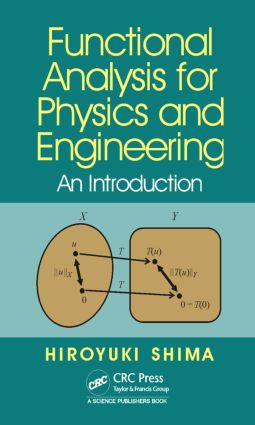# Functional Analysis for Physics and Engineering

## An Introduction, 1st Edition

CRC Press

285 pages | 96 B/W Illus.

##### Purchasing Options:\$ = USD
Hardback: 9781482223019
pub: 2015-12-23
SAVE ~\$33.00
\$165.00
\$132.00
x
eBook (VitalSource) : 9780429068164
pub: 2016-01-05
from \$28.98

FREE Standard Shipping!

### Description

This book provides an introduction to functional analysis for non-experts in mathematics. As such, it is distinct from most other books on the subject that are intended for mathematicians. Concepts are explained concisely with visual materials, making it accessible for those unfamiliar with graduate-level mathematics. Topics include topology, vector spaces, tensor spaces, Lebesgue integrals, and operators, to name a few. Two central issues—the theory of Hilbert space and the operator theory—and how they relate to quantum physics are covered extensively. Each chapter explains, concisely, the purpose of the specific topic and the benefit of understanding it. Researchers and graduate students in physics, mechanical engineering, and information science will benefit from this view of functional analysis.

### Table of Contents

Prologue

What Functional Analysis tells us

From perspective of the limit

From perspective of infinite dimension

From perspective of quantum mechanical theory

Topology

Fundamentals

Continuous mapping

Homeomorphism

Vector space

What is vector space?

Property of vector space

Hierarchy of vector space

Hilbert space

Basis and completeness

Equivalence of L2 spaces with 2 spaces

Tensor space

Two faces of one tensor

"Vector" as a linear function

Tensor as a multilinear function

Component of tensor

Lebesgue integral

Motivation & Merits

Measure theory

Lebesgue integral

Lebesgue convergence theorem

Lp space

Wavelet

Continuous wavelet analysis

Discrete wavelet analysis

Wavelet space

Distribution

Motivation & Merits

Establishing the concept of distribution

Examples of distribution

Mathematical manipulation of distribution

Completion

Completion of number space

Sobolev space

Operator

Classification of operators

Essence of operator theory

Preparation toward eigenvalue-like problem

Practical importance of non-continuous operators

Real number sequence

A.1 Convergence of real sequence

A.2 Bounded sequence

A.3 Uniqueness of the limit of real sequence

Cauchy sequence

B.1 What is Cauchy sequence?

B.2 Cauchy criterion for real number sequence

Real number series

C.1 Limit of real number series

C.2 Cauchy criterion for real number series

Continuity and smoothness of function

D.1 Limit of function

D.2 Continuity of function

D.3 Derivative of function

D.4 Smooth function

Function sequence

E.1 Pointwise convergence

E.2 Uniform convergence

E.3 Cauchy criterion for function series

F Uniformly convergent sequence of functions

F.1 Continuity of the limit function

F.2 Integrability of the limit function

F.3 Differentiability of the limit function

G Function series

G.1 Infinite series of functions

G.2 Properties of uniformly convergent series of functions

H Matrix eigenvalue problem

H.1 Eigenvalue and eigenvector

H.2 Hermite matrix

I Eigenspace decomposition

I.1 Eigenspace of matrix

I.2 Direct sum decomposition

Index

### Subject Categories

##### BISAC Subject Codes/Headings:
MAT003000
MATHEMATICS / Applied
MAT004000
MATHEMATICS / Arithmetic
MAT037000
MATHEMATICS / Functional Analysis